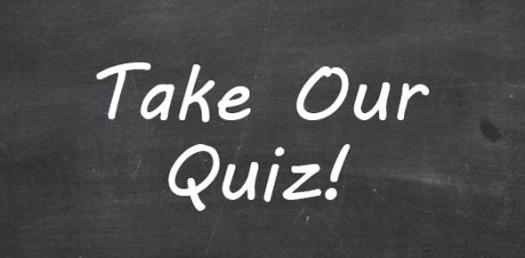# Hyphens With Compound Adjectives Quiz

10 Questions | Attempts: 305Settings.

• 1.
Is the use of a hyphen correct?8 week money back guarantee
• A.

True

• B.

False

• 2.
Which one below is correct?
• A.

Tight-fitting

• B.

Tight fitting

• 3.
Which one below is correct?
• A.

Non refundable

• B.

Non-refundable

• 4.
Which one below is correct?
• A.

James is a second rate plumber.

• B.

James is a second-rate plumber.

• 5.
Is the use of a hyphen correct?air-tight
• A.

True

• B.

False

• 6.
Which one below is correct?
• A.

A heavy metal-detector

• B.

A heavy-metal detector

• 7.
Is the use of a hyphen correct?a free-and-easy relationship
• A.

True

• B.

False

• 8.
Is the use of a hyphen correct?new-born-baby
• A.

True

• B.

False

• 9.
Which one below is correct?
• A.

• B.Back to top